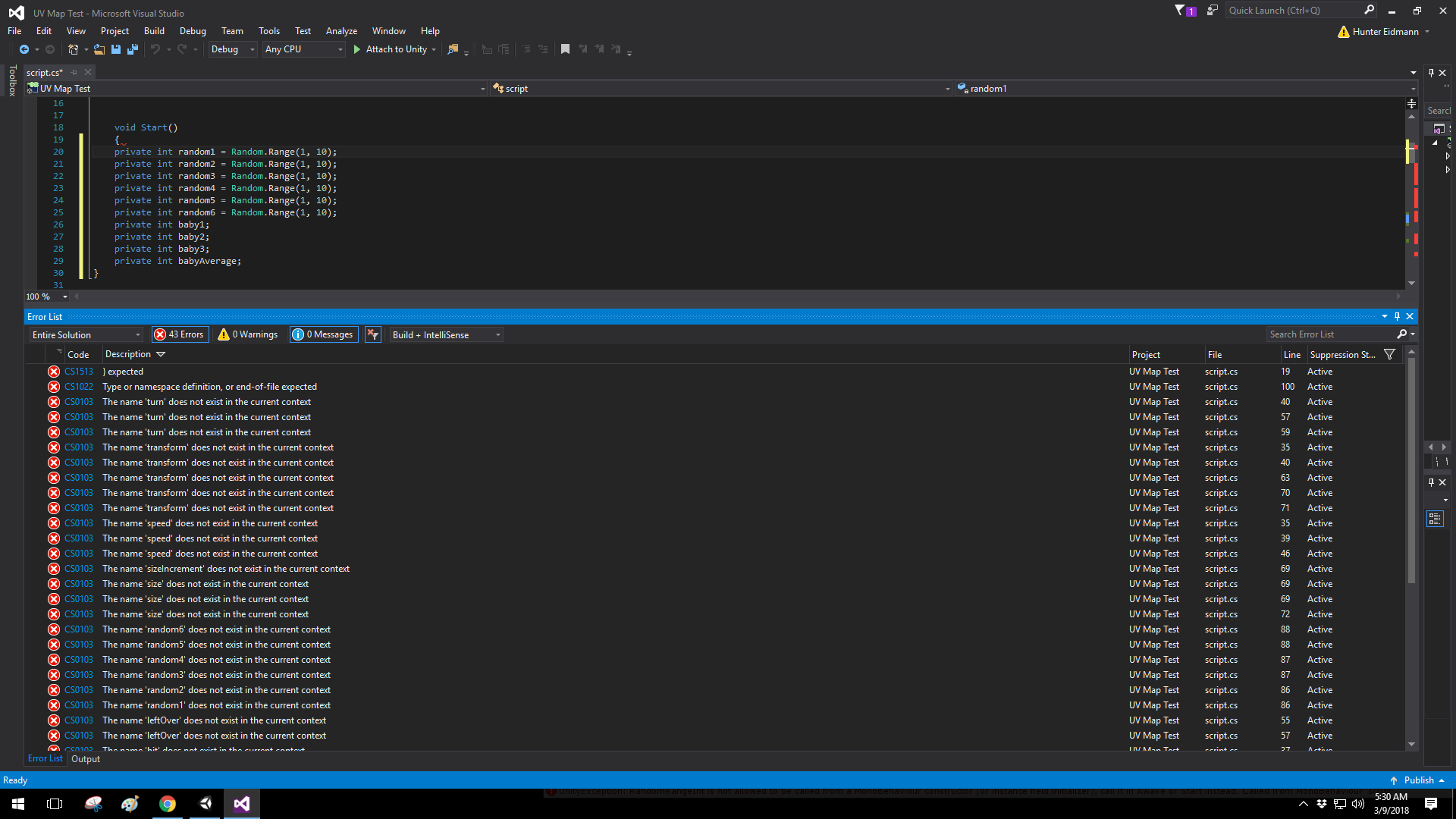## Assigning Random.Range to a variable

I have assigned Random.Range to multiple variables above the Start function as shown below and I get this error, "RandomRangeInt is not allowed to be called from a MonoBehaviour constructor (or instance field initializer), call it in Awake or Start instead. "

So I tried cutting and pasting all these variables into the Start function and got a deluge of errors seen in the picture. I am not sure how to resolve this and the code works perfectly fine notwithstanding.

private int random1 = Random.Range(1, 10);
private int random2 = Random.Range(1, 10);
private int random3 = Random.Range(1, 10);
private int random4 = Random.Range(1, 10);
private int random5 = Random.Range(1, 10);
private int random6 = Random.Range(1, 10);• You have to remove "private" from the variables in the Start method. Also if you just use them within the Start method you can't use them in any other methods outside of that, they would be considered local variables to that method. Instead you should declare them at the top of your class then assign the value in the Start method like this:

```using System.Collections;
using System.Collections.Generic;
using UnityEngine;
public class Num : MonoBehaviour {
private int random1;
private int random2;
private int random3;
private int random4;
private int random5;
private int random6;
// Use this for initialization
void Start () {
random1 = Random.Range(1, 10);
random2 = Random.Range(1, 10);
random3 = Random.Range(1, 10);
random4 = Random.Range(1, 10);
random5 = Random.Range(1, 10);
random6 = Random.Range(1, 10);

}

}```

Another thing I will mention, since each variable is basically doing the exact same thing it might be easier and more efficient to use one variable. If you wanted to change the value of it randomly you could put it into a method that you can call every time you want to use that variable. Something like this:

```public randomValue;
void Start ()
{
randomValue = Random.Range(1,10);
}

void RandomVariableChange ()
{
randomValue = Random.Range(1,10);
}```

or you could just use

`randomValue = Random.Range(1,10)`

every time you go to use it so the value changes

This will give this variable a random number when you first start it, then the "RandomVariableChange" method can be called to change the value to something different. That is assuming that you just want to use this one at a time and not a whole set of random numbers at once.